Courses

# Test: Fluids- 2

## 30 Questions MCQ Test Physics Class 11 | Test: Fluids- 2

Description
This mock test of Test: Fluids- 2 for JEE helps you for every JEE entrance exam. This contains 30 Multiple Choice Questions for JEE Test: Fluids- 2 (mcq) to study with solutions a complete question bank. The solved questions answers in this Test: Fluids- 2 quiz give you a good mix of easy questions and tough questions. JEE students definitely take this Test: Fluids- 2 exercise for a better result in the exam. You can find other Test: Fluids- 2 extra questions, long questions & short questions for JEE on EduRev as well by searching above.
QUESTION: 1

### As a bubble comes from the bottom of a lake to the top, its radius

Solution:

PV = constant

As a bubble comes from bottom to top , the pressure decrease from ( P0 + ρgh )  to P0

∴  Volume increases and hence radius increases.

QUESTION: 2

### To get the maximum flight, a ball must be thrown as

Solution:

Velocity of ball at top = V1 = VT + VCOM

Velocity of ball at bottom = V2 = | VT – VCOM |

V1 >> V2 than other option.

∴ More pressure difference is created because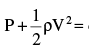constant

QUESTION: 3

### The tube shown is of uniform cross-section. Liquid flows through it at a constant speed in the direction shown by the arrows. The liquid exerts on the tube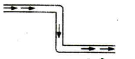Solution:

The forces exerted by the liquid at the bends are shown. (The liquid undergoes change of momentum only at these points, and hence the liquid and tube exert forces on each other.) The two forces form a couple exerting a clockwise torque.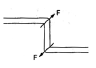QUESTION: 4

Water coming out of the mouth of a tap and falling vertically in streamline flow forms a tapering column, i.e., the area of cross-section of the liquid column decreases as it moves down. Which of the following is the most accurate explanation for this?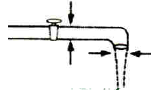Solution:
QUESTION: 5

A cylindrical drum, open at the top, contains 30 litres of water. It drains out through a small opening at the bottom.10 litre of water comes out in time t, the next 10 litres in a further time t2 and the last 10 litres in a further time t3.Then

Solution:

We know that,
Velocity of efflux is given as v = √2gh
As the water level in the tank decreases, the velocity with which water comes out from the opening decreases due to decrease in pressure with height. Hence, the time taken to empty the tank increases with decrease in velocity and height of water level.
Hence C is the correct answer.

QUESTION: 6

The valve V in the bent tube is initially kept closed. Two soap bubbles A (smaller) and B (larger) are formed at the two open ends of the tube. V is now opened, and air can flow freely between the bubbles.

Solution:
QUESTION: 7

Water rises in a straight capillary tube upto a height of 5 cm when held vertical in water. If the tube is bent as shown in figure then the height of water column in it will be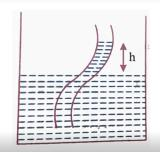Solution:
QUESTION: 8

A piece of cork starts from rest at the bottom of a lake and floats up. Its velocity v is plotted against time t. Which of the following best represents the resulting curve?

Solution:

As the cork moves up, the force due to buoyancy remains constant. As its speed increases, the retarding force due to viscosity increases, being proportional to the speed. Thus. the acceleration gradually decreases. The acceleration is variable, and hence the relation between velocity and time is not linear.

QUESTION: 9

When cooking oil is heated in frying pan, the oil moves around in the pan more easily when it is hot. The main reason for this is that with rise in temperature, there is a decrease in

Solution:
QUESTION: 10

A tank with vertical walls is mounted so that its base is at a height H above the horizontal ground. The tank is filled with water to the depth h. A hole is punched in the side wall of the tank at a depth x below the water surface. To have maximum range of the emerging stream, the value of x is

Solution:

To have the maximum range of the emerging stream, the value of x is to be (h/2) where h is the depth of water.

QUESTION: 11

The work done in increasing the volume of a soap bubble of radius r to 27 times will be, if the surface tension of soap solution is T

Solution: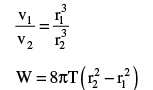QUESTION: 12

The excess pressure inside first soap bubble is 3 times that inside the second bubble. Then the ratio of volumes of the first and the second bubbles is

Solution: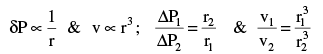QUESTION: 13

Water is rises to a height h1 in a capillary tube in a stationary lift. If the lift moves up with uniform acceleration it rises to a height h2, then acceleration of the lift is

Solution:

h2 (g + a) = h1 g

QUESTION: 14

A ball of mass ‘m’ and radius ‘r’ is released in viscous liquid. The value of its terminal velocity is proportional to

Solution: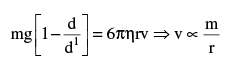QUESTION: 15

Viscosity is the property by virtue of which a liquid

Solution:
QUESTION: 16

For an ideal fluid, viscosity is

Solution:

A fluid that has no resistance to shear stress is known as an ideal fluid.

QUESTION: 17

A liquid of density ρ and coefficient of viscosity η, flows with velocity v through a tube of diameter D. A quantity R = ρVD/ η determines whether the flow will be streamlined or turbulent. R has the dimension of

Solution:
QUESTION: 18

Coefficient of viscosity of a gas

Solution:
QUESTION: 19

Water flows through a frictionless duct with a cross-section varying as shown in figure. Pressure P at points along the axis is represented by (Assume water to be non-viscous)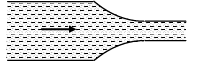Solution:

Application of Bernoulli’s theorem larger area of cross section implies less velocity which in turn implies higher pressure.

QUESTION: 20

There are two identical small holes on the opposite sides of a tank containing a liquid. The tank is open at the top. The difference in height between the two holes is h. As the liquid comes out of the two holes, the tank will experience a net horizontal force proportional to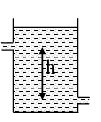Solution: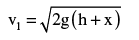Let ρ = density of the liquid.

Let α = area of a cross-section of each hole.

Volume of liquid discharged per second at a hole = αv.

Mass of liquid discharged per second = αvρ.

Momentum of liquid discharged per second = αv2ρ.

∴ the force exerted at the upper hole (to the right) = αρv22

and  the force exerted at the lower hole (to the left) = αρv12

Net force on the tank = 2αρgh.

QUESTION: 21

n drops of liquid, each with surface energy E, join to form a single drop. In this process

Solution: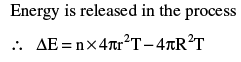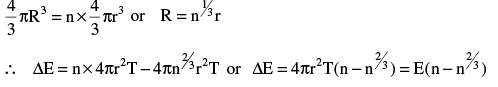QUESTION: 22

Two spherical soap bubbles of radii R1 and R2 combine under isothermal condition to form a single bubble. The radius of the resultant bubble is

Solution: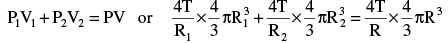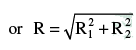QUESTION: 23

For the arrangement shown in figure, the time interval after which the water jet ceases to cross the wall (area of cross section of tank is A and orifice is ‘a’)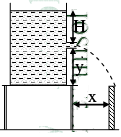Solution:

Velocity of efflux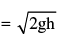Find ‘h’ to have range of ejected water ≥ x x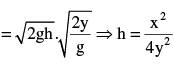QUESTION: 24

Two soap bubbles with radii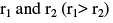come in contact. Their common surface has a radius of curvature r. Then

Solution:

Let p0 = atmospheric pressure,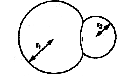p1 and p2 = pressures inside the two bubbles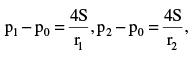Let r = radius of curvature of the common surface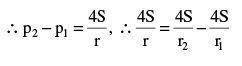QUESTION: 25

A constant height of 20 cm maintained in the contains water of 1 kg as shown in figure. A small orifice area 10-2 m2 is made at bottom to the vertical wall of container the ejected water is directed as shown in figure. Assuming mass of container is negligible, the net force on container is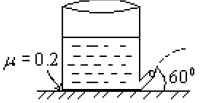Solution:

FBD of container: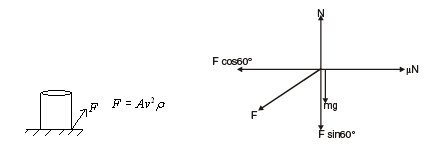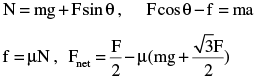QUESTION: 26

The surface of water in a water tank on the top of a house is 4 m above the tap level. The pressure of water at the tap when the tap is closed is (Density of water = 1000 kg/m3 g = 10 m/s2

Solution: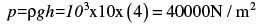QUESTION: 27

Water flows through a horizontal tube as shown in figure. If the difference of heights of water column in the vertical tubes is 60 m, and the areas areas of cross-section at A and B are 4m2 and 2 m2 respectively , find the rate of flow of water across any section is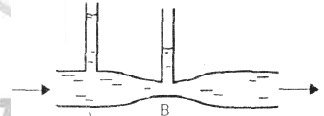Solution:

Using the following relations,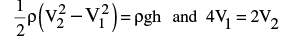We get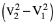= 2gh = 1200 and V2 = 2V1
Therefore, V1 = 20, V2 = 40m/s and the rate of flow of water = 80 m3/s

QUESTION: 28

A tube has two area of cross-sections as shown in figure. The diameters of the tube are 8 mm and 2 mm. Find range of water falling on horizontal surface, if piston is moving with a constant velocity of 0.25m/s, h = 1.25 m (g = 10 m/s2)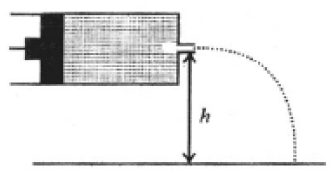Solution: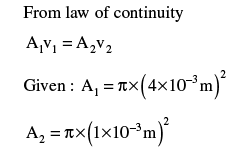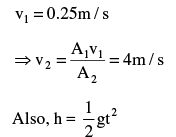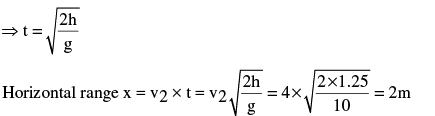QUESTION: 29

A large open tank has two holes in the wall. One is a square hole of side L at a depth y from the top and the other is a circular hole of radius R at a depth 4y from the top. When the tank is completely filled with water, the quantities of water lowing out per second  from both holes are the same. Then R is equal to

Solution: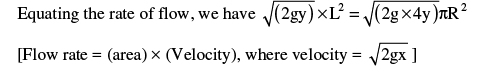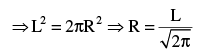QUESTION: 30

Water from a tap emerges vertically downwards with an initial speed of  1.0 m/s. The cross sectional area of the tap is 10-4 m2.Assume that the pressure is constant throughout the steam of water, and that the flow is steady. The cross sectional area of the stream 0.15 m below the tap is

Solution:

The equation of continuity is :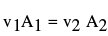where v and A represent the speed of water stream and its area of cross section, respectively. We are given that,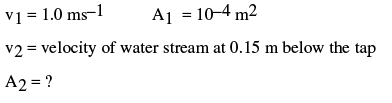For calculating v2